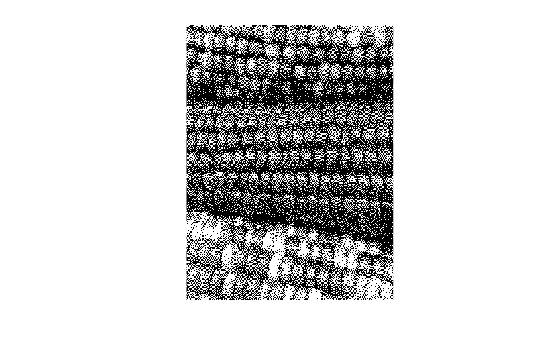# dither

Convert image, increasing apparent color resolution by dithering

## Syntax

``X = dither(RGB,map)``
``X = dither(RGB,map,Qm,Qe)``
``BW = dither(I)``

## Description

````X = dither(RGB,map)` creates an indexed image approximation of the RGB image by dithering the colors in the colormap `map`.```
````X = dither(RGB,map,Qm,Qe)` also specifies the number `Qm` of quantization bits to use along each color axis for the inverse colormap, and the number `Qe` of quantization bits to use for the color space error calculations.```

example

````BW = dither(I)` converts the grayscale image `I` to the binary (black and white) image `BW` by dithering.```

## Examples

collapse all

Read the grayscale image from the `corn.tif` file into the MATLAB® workspace. The grayscale version of the image is the third image in the file.

`corn_gray = imread('corn.tif',3);`

Display the grayscale image using `imshow`.

`imshow(corn_gray)`Convert the image to binary by using the `dither` function.

`corn_bw = dither(corn_gray);`

Display the binary image. Although pixels in the binary image only have the value `0` or `1`, the image appears to have shades of gray because of dithering.

`imshow(corn_bw)`## Input Arguments

collapse all

RGB image, specified as an m-by-n-by-3 array of nonnegative numbers. If you specify an image of data type `double`, then values must be in the range [0, 1].

Data Types: `single` | `double` | `uint8` | `uint16`

Input colormap, specified as a c-by-3 matrix with values in the range [0, 1]. Each row of `map` is a three-element RGB triplet that specifies the red, green, and blue components of a single color of the colormap. The colormap has a maximum of 65,536 colors.

Data Types: `double`

Quantization bits along each color axis for the inverse colormap, specified as a positive integer. If `Qe` is less than `Qm`, then dithering cannot be performed and the `dither` function returns an undithered indexed image in `X`.

Data Types: `double`

Quantization bits for the color space error calculations, specified as a positive integer. If `Qe` is less than `Qm`, then dithering cannot be performed and the `dither` function returns an undithered indexed image in `X`.

Data Types: `double`

Grayscale image, specified as an m-by-n matrix of nonnegative numbers. If you specify an image of data type `double`, then values must be in the range [0, 1].

Data Types: `single` | `double` | `int16` | `uint8` | `uint16`

## Output Arguments

collapse all

Indexed image, returned as an m-by-n matrix of nonnegative integers. If the length of `map` is less than or equal to 256, then the output image is of class `uint8`. Otherwise, the output image is of class `uint16`. The value `0` in the output array `X` corresponds to the first color in the colormap.

Note

The values in image `X` are indexes into the colormap `map` and should not be used in mathematical processing, such as filtering operations.

Data Types: `uint8` | `uint16`

Binary image, returned as an m-by-n logical matrix.

Data Types: `logical`

## Algorithms

`dither` increases the apparent color resolution of an image by applying Floyd-Steinberg's error diffusion dither algorithm .

 Floyd, R. W., and L. Steinberg, "An Adaptive Algorithm for Spatial Gray Scale," International Symposium Digest of Technical Papers, Society for Information Displays, 1975, p. 36.

 Lim, Jae S., Two-Dimensional Signal and Image Processing, Englewood Cliffs, NJ, Prentice Hall, 1990, pp. 469–476.